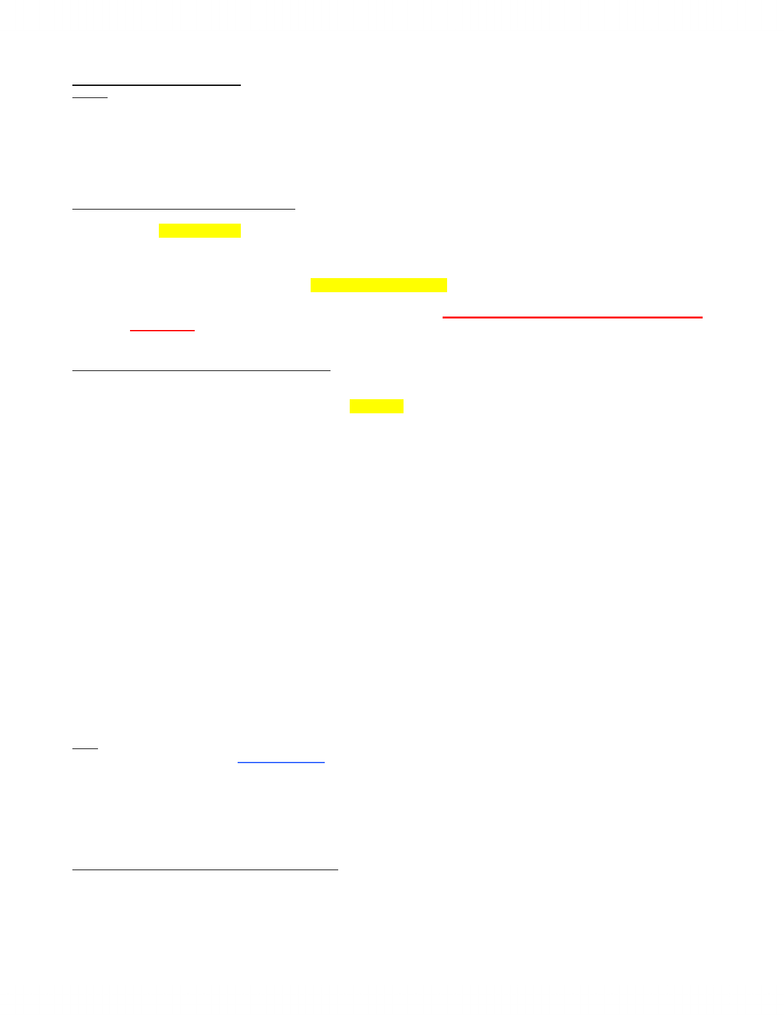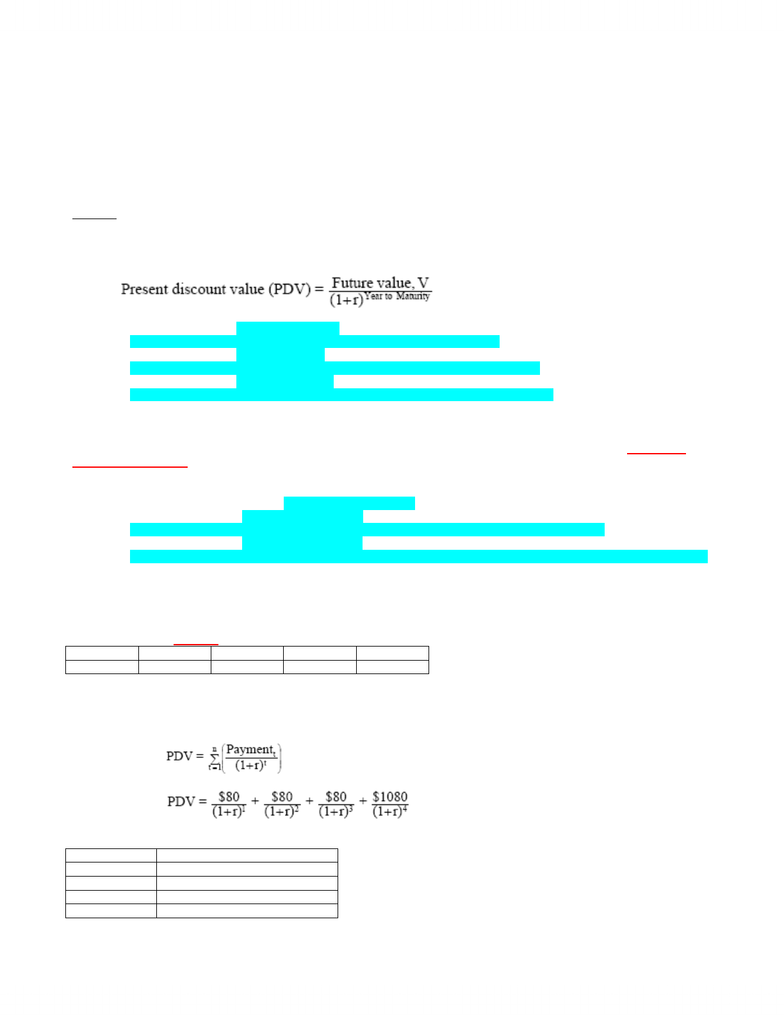# MGEA06H3 Lecture Notes - Market Liquidity, Soo Line Railroad, Shortage

39 views5 pagesMONEY AND INTEREST RATE
Outline
x A brief review—why we want to hold money?
x The cost of holding money and the demand for money.
x The relationship between bond prices and interest rates.
x The inverse relationship between interest rate and investment—a revisit.
x The relationship between money supply, interest rate, and investment.
x How does the central bank affect the money supply?
A Brief ReviewWhy We Want to Hold Money?
What “Money Means in Economics
x Money is the most liquid asset in our economy, an asset is said to be very liquid if it can easily be converted into goods and services
x Obviously, there are other ways to store value (i.e., hold our wealth in other forms of assets) that are not so liquid.
o Examples of illiquid assets include real estate, cars and etc.
x To some extent, the choice to hold money is a choice among different assets (calledportfolio choices”).
o We ask the question: What is the portion of our wealth that we want to hold in form of liquid asset (money)?
o In economics, the phrase “we have a lot of money means we have chosen to hold a larger portion of our wealth in the form
of liquid asset.
o For everyone else, the phrase “we have a lot of money” means we are wealthy.
The Cost of Holding Money and the Demand for Money
The Cost of Holding Money
x Question: What is the opportunity cost of holding money?
x Answer: The opportunity cost of holding money is the interest rate. Why?
o Recall, to some extent, the choice to hold money is a choice among different assets.
o When we decide to hold our wealth in form of money, we give up the opportunity to hold our wealth in other form of assets.
o The value of money DOES NOT “grow” and give us any interest. (Think about what happens if you put a \$100 bill under
your pillow tonight and then take it out a year later; it is the same \$100.)
o However, other assets such as bonds give us a return such as interest.
o Therefore, the opportunity cost of holding money is the interest rate (the rate of return we could have earned if we hold our
wealth in the form of bonds and etc.).
x Question: What happens to the cost of holding money when interest rate rises?
x Answer: When interest rate increases, cost of holding money increases.
o We hold less money and more other interest-bearing assets.
o Demand for money decreases
The Demand for Money
x The demand for money (MD) depends on:
1) Income, Y
o We hold money as a means to facilitate our daily purchases.
o Holding all else constant, when Y increases, our purchases of goods and services increases Æ demand for money increases.
o There is a positive relationship Y and MD.
2) Interest rate, r
o Holding all else constant, when r increases, cost of holding money increases Æ demand for money decreases.
o There is a negative relationship between r and MD.
x In notation form:
MD = demand for liquidity = L (r, Y)
L is the liquidity function
It represents all liquidity for money
Note:
x In economics, we also call the demand for money as a demand for liquidity because money is the most liquid asset in the economy.
If we want to hold more money, this means we want to hold more liquid assets.
x In real life the decision of holding money is more complicated. Why?
o Money also serves as a store of value.
o However, if the assets become less liquid (i.e., it takes a longer time to be converted into goods and services), the rates of
return of these assets tend to rise because investors need higher returns to compensate for the fact that the assets cannot be
The Relationship between Bond Prices and Interest Rates
Assumptions:
1) There are only two assets in the economy: cash and bonds.
2) Money (cash) does not give any returns to its holders.
3) Bonds give a return to its holders but it is not liquid as cash.
x Based on these assumptions, we know that the choice of holding money is the choice between holding cash and holding bonds.
www.notesolution.com
Unlock document

This preview shows pages 1-2 of the document.
Unlock all 5 pages and 3 million more documents.x Question: What is a bond?
x Answer: A bond is a piece of paper with
o A face value
o A maturity date
o A coupon rate—the stated rate of interest on the face of the bond
* IMPORTANT: A typical bond is NOT the Canada Savings Bond.
o Canada Savings Bond pays regular coupon payments and can be cashed in at any time.
o Most bonds CANNOT be cashed in before maturity date.
o Although we cannot cash in the bond, we can sell it!
A One-Year Bond
Example: A bond with a face value of \$100, a coupon rate of 5%, and matures in one year.
x This means the bond promises to its holder \$105 in one year (\$100 face value + \$5 coupon).
x Question: At the beginning of the year, what is the price of this bond? Or, how much would it cost for us to buy this bond at the
beginning of the year?
x Answer: It depends on the interest rate (r).
FV = \$105 Year to Maturity = 1
o If r = 2%, then PDV = \$105 / 1.02 = \$102.94
Means that to get \$105 in a year at 5%, \$102.94 needs to be invested right now.
o If r = 5%, then PDV = \$105 / 1.05 = \$100
Means that to get \$105 in a year at 5%, \$100 needs to be invested in the bond right now.
o If r = 8%, then PDV = \$105 / 1.08 = \$97.22
Means that to get \$105 in a year at 5%, \$97.22 needs to be paid out for the bond right now.
x Observation: When r increases, PDV of this one-year bond decreases Æ the amount we paid to get this bond decreases (i.e., the
price of this one-year bond decreases).
Multi-Year Bond Without Coupon Payment
Suppose we have a compounding bond paying off at 5% at the end of 10 years and the face value is \$100 (i.e., there are no payments
between Year 1 – Year 9).
x Question: What is the price of this 10-year bond?
o Value of the bonds in 10 years = \$100 (1 + 0.05)10 = \$162.89
o If r = 10%, then PDV = \$162.89 / (1.1)10 = \$62.80
This means that if the future value of the bond is \$162.89, then only \$62.80 needs to be paid right now.
o If r = 20%, then PDV = \$162.89 / (1.2)10 = \$26.31
If interest rate shoots up to 20%, then only \$26.31 needs to be paid for the bond right now to get \$162.89 10 years from now.
x Observation: When r increases, PDV of bond decreases Æ the price of the bond decreases.
Multi-Year Bond With Coupon Payments
x The pricing of bonds gets complicated when the bond makes coupon payments.
Suppose a bond with a face value of \$1000, a coupon rate of 8%, and a maturity of 4 years.
x This bond will make a coupon payment at the end of each year until it matures.
x Let’s look at the cash flow of this 4-year bond:
In 1 year In 2 years In 3 years In 4 years
Cash flow \$80 \$80 \$80 \$1080
x Question: What is the PDV of this bond? Or, what is the price of this bond?
x Answer: To price a bond, we need the following:
o The promised payment stream.
o The interest rate to discount these promised payments.
o The price of the bond = the present value of these promised payments.
o In our example, the price of the bond:
In real life, r changes so the PDV changes every year.
o The PDV at different interest rates:
Interest rate, r PDV of bond = bond price
4% (r = 0.04) PDV = \$1145.20
6% (r = 0.06) PDV = \$1069.30
8% (r = 0.08) PDV = \$1000
10% (r = 0.1) PDV = \$936.60
o When r increases, PDV decreases Æ price of the bond decreases.
www.notesolution.com
Unlock document

This preview shows pages 1-2 of the document.
Unlock all 5 pages and 3 million more documents.

## Document Summary

The cost of holding money and the demand for money. The relationship between bond prices and interest rates. The inverse relationship between interest rate and investment a revisit. The relationship between money supply, interest rate, and investment. N money is the most liquid asset in our economy, an asset is said to be very liquid if it can easily be converted into goods and services (i. e. , easy to buy stuffs). N obviously, there are other ways to store value (i. e. , hold our wealth in other forms of assets) that are not so liquid: examples of illiquid assets include real estate, cars and etc. In economics, the phrase we have a lot of money means we have chosen to hold a larger portion of our wealth in the form of liquid asset. For everyone else, the phrase we have a lot of money means we are wealthy. N answer: the opportunity cost of holding money is the interest rate.

## Get access

\$10 USD/m
Billed \$120 USD annuallyHomework Help
Study Guides
Textbook Solutions
Class Notes
Textbook Notes
Booster Class